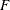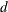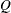## Getting Started: NETFLOW Procedure

To solve network programming problems with side constraints using PROC NETFLOW, you save a representation of the network and the side constraints in three SAS data sets. These data sets are then passed to PROC NETFLOW for solution. There are various forms that a problem’s data can take. You can use any one or a combination of several of these forms.

The NODEDATA= data set contains the names of the supply and demand nodes and the supply or demand associated with each. These are the elements in the column vectorin problem (NPSC).

The ARCDATA= data set contains information about the variables of the problem. Usually these are arcs, but there can also be data related to nonarc variables in the ARCDATA= data set.

An arc is identified by the names of its tail node (where it originates) and head node (where it is directed). Each observation can be used to identify an arc in the network and, optionally, the cost per flow unit across the arc, the arc’s capacity, lower flow bound, and name. These data are associated with the matrixand the vectors,, andin problem (NPSC).

Note: Althoughis a node-arc incidence matrix, it is specified in the ARCDATA= data set by arc definitions.

In addition, the ARCDATA= data set can be used to specify information about nonarc variables, including objective function coefficients, lower and upper value bounds, and names. These data are the elements of the vectors,, andin problem ( NPSC). Data for an arc or nonarc variable can be given in more than one observation.

Supply and demand data also can be specified in the ARCDATA= data set. In such a case, the NODEDATA= data set may not be needed.

The CONDATA= data set describes the side constraints and their right-hand sides. These data are elements of the matricesandand the vector. Constraint types are also specified in the CONDATA= data set. You can include in this data set upper bound values or capacities, lower flow or value bounds, and costs or objective function coefficients. It is possible to give all information about some or all nonarc variables in the CONDATA= data set.

An arc is identified in this data set by its name. If you specify an arc’s name in the ARCDATA= data set, then this name is used to associate data in the CONDATA= data set with that arc. Each arc also has a default name that is the name of the tail and head node of the arc concatenated together and separated by an underscore character; `tail_head`, for example.

If you use the dense side constraint input format (described in the section CONDATA= Data Set) and want to use the default arc names, these arc names are names of SAS variables in the VAR list of the CONDATA= data set.

If you use the sparse side constraint input format (see the section CONDATA= Data Set) and want to use the default arc names, these arc names are values of the COLUMN list SAS variable of the CONDATA= data set.

The execution of PROC NETFLOW has three stages. In the preliminary (zeroth) stage, the data are read from the NODEDATA= data set, the ARCDATA= data set, and the CONDATA= data set. Error checking is performed, and an initial basic feasible solution is found. If an unconstrained solution warm start is being used, then an initial basic feasible solution is obtained by reading additional data containing that information in the NODEDATA= data set and the ARCDATA= data set. In this case, only constraint data and nonarc variable data are read from the CONDATA= data set.

In the first stage, an optimal solution to the network flow problem neglecting any side constraints is found. The primal and dual solutions for this relaxed problem can be saved in the ARCOUT= data set and the NODEOUT= data set, respectively. These data sets are named in the PROC NETFLOW, RESET, and SAVE statements.

In the second stage, an optimal solution to the network flow problem with side constraints is found. The primal and dual solutions for this side constrained problem are saved in the CONOUT= data set and the DUALOUT= data set, respectively. These data sets are also named in the PROC NETFLOW, RESET, and SAVE statements.

If a constrained solution warm start is being used, PROC NETFLOW does not perform the zeroth and first stages. This warm start can be obtained by reading basis data containing additional information in the NODEDATA= data set (also called the DUALIN= data set) and the ARCDATA= data set.

If warm starts are to be used in future optimizations, the FUTURE1 and FUTURE2 options must be used in addition to specifying names for the data sets that contain the primal and dual solutions in stages one and two. Then, most of the information necessary for restarting problems is available in the output data sets containing the primal and dual solutions of both the relaxed and side constrained network programs.